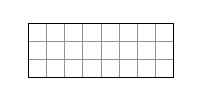### Home > AC > Chapter 1 > Lesson 1.1.1 > Problem1-5

1-5.

The area of the rectangle below is $24$ square units. On graph paper, draw and label all possible rectangles with an area of $24$ square units. Use only whole numbers for the dimensions (measurements).$\text{Area}=24\;\text{square units}$

$1$ by $24$, $2$ by $12$, $3$ by $8$, and $4$ by $6$

1. Find the perimeter of each of these rectangles. You may want to refer to the Math Notes box for this lesson for more information on the perimeter of a figure.

The perimeter is the distance around the outside of a figure.

$50$, $28$, $22$, and $20$ units

2. Of these rectangles, which has the largest perimeter? Which has the smallest perimeter? Describe these shapes. Remember to use complete sentences.

The largest perimeter is a thin rectangle, P = $50$ units;
the smallest is close to a square shape, P = $20$ units.